Chapter 13 Class 12 Probability

Class 12
Important Questions for exams Class 12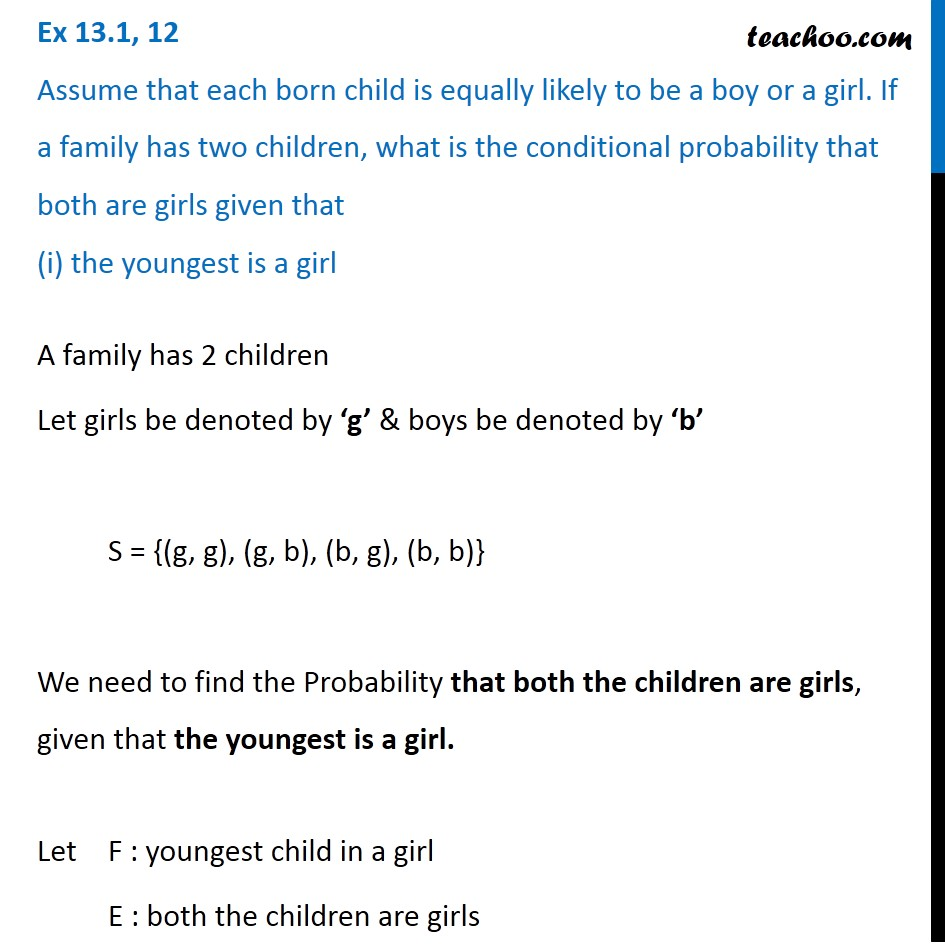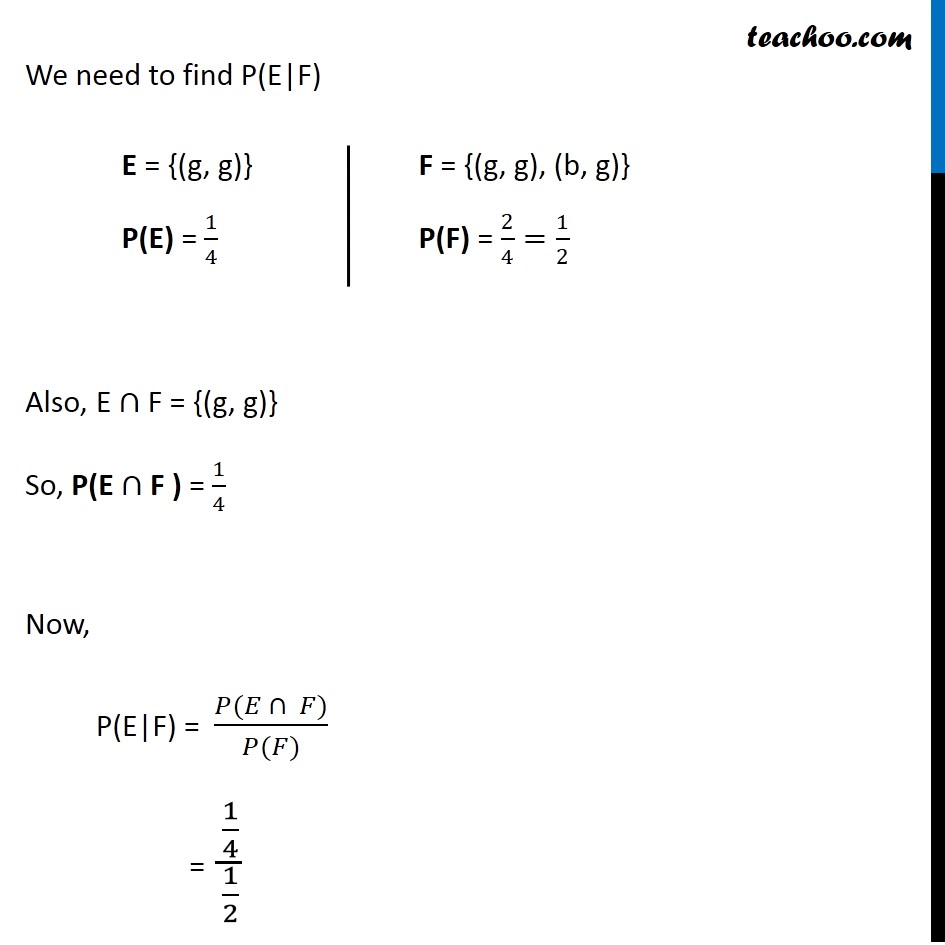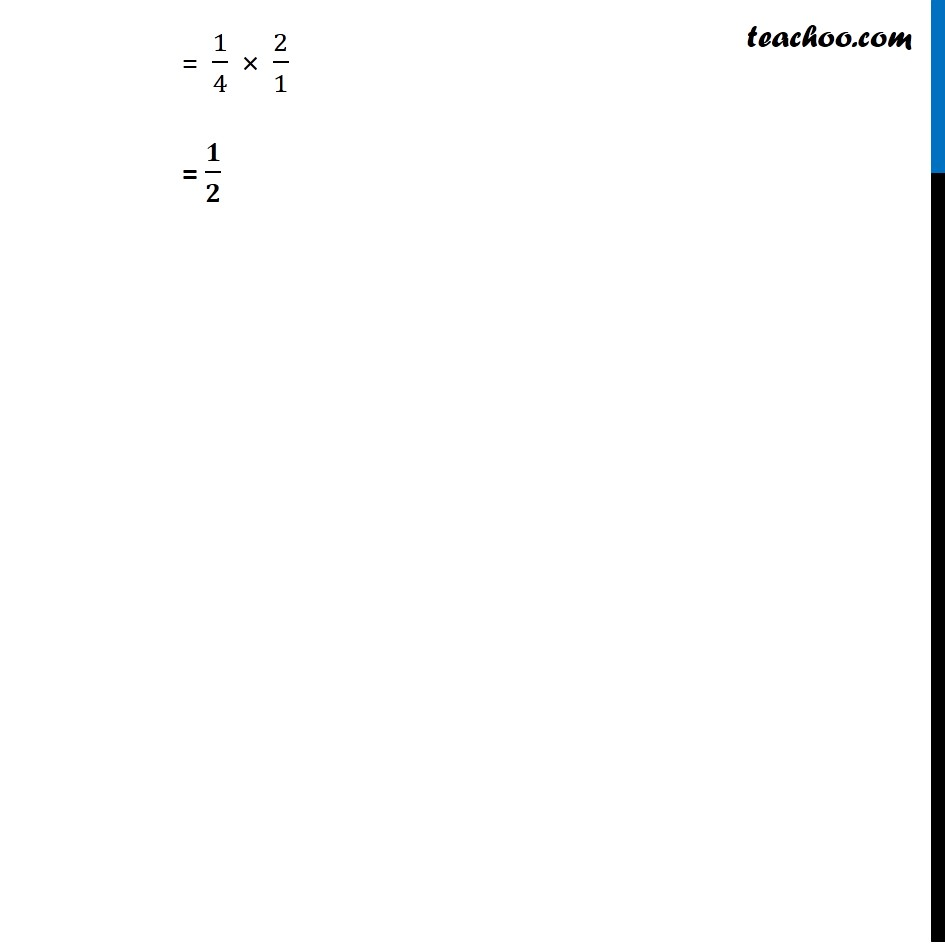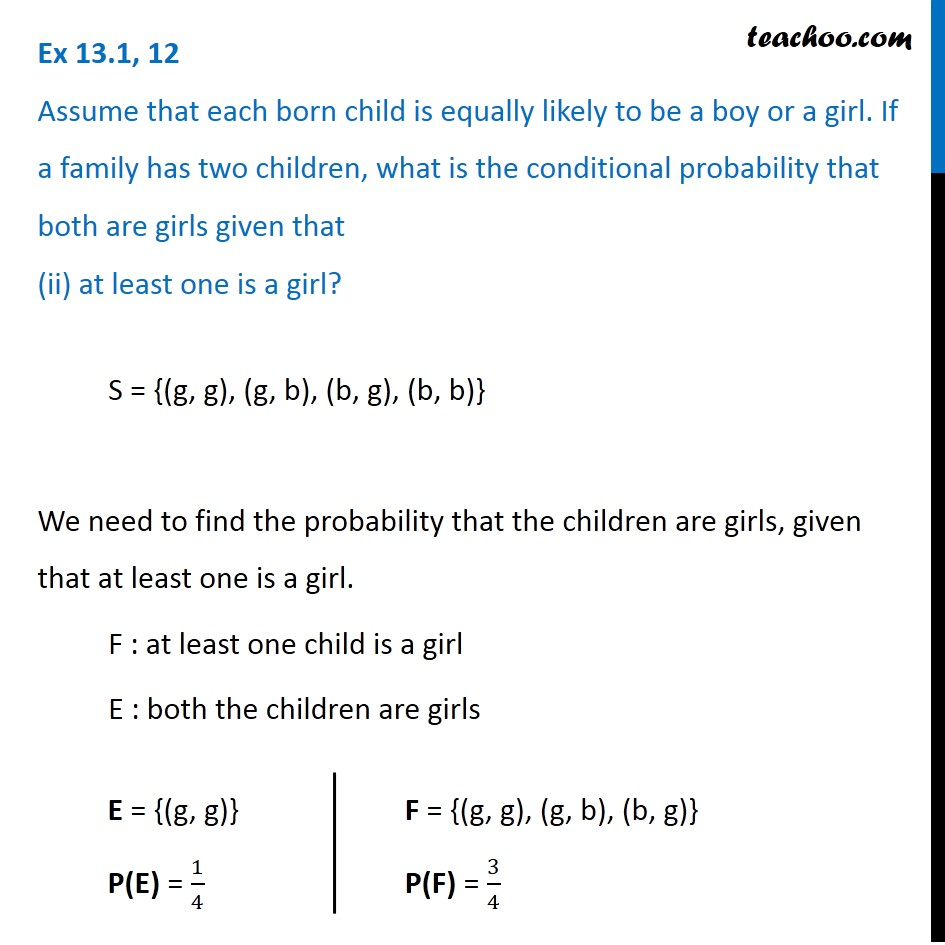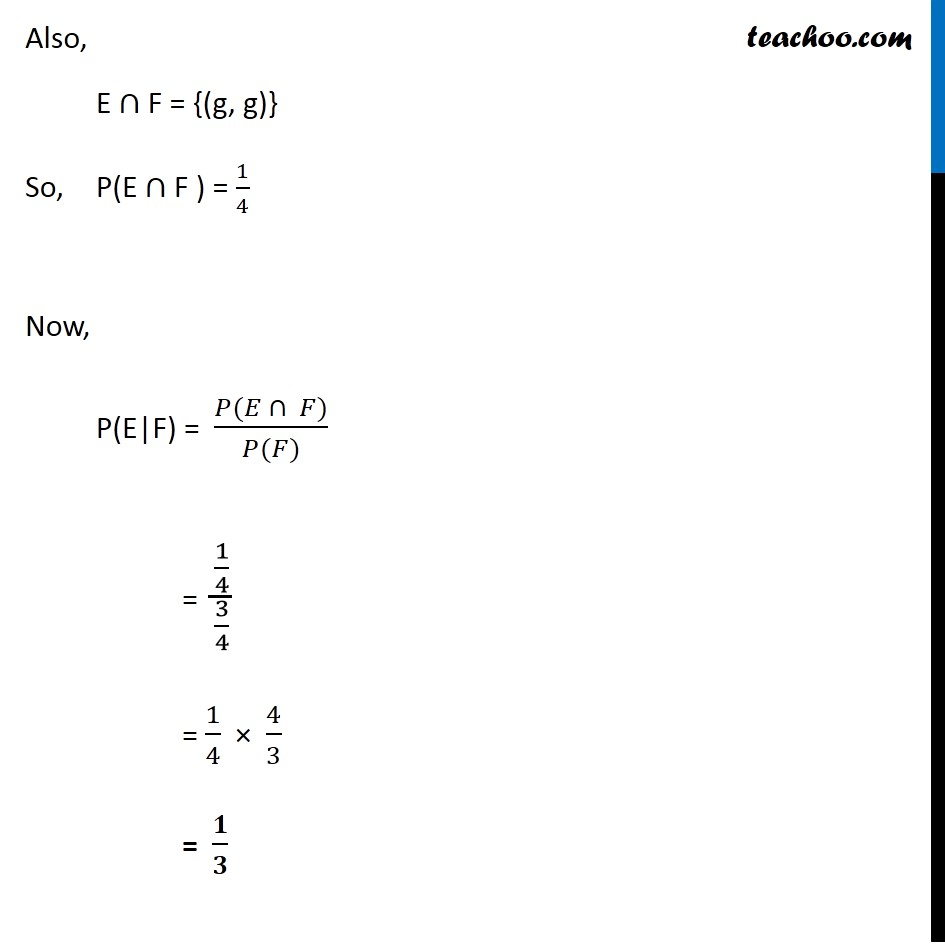Learn in your speed, with individual attention - Teachoo Maths 1-on-1 Class

### Transcript

Ex 13.1, 12 Assume that each born child is equally likely to be a boy or a girl. If a family has two children, what is the conditional probability that both are girls given that (i) the youngest is a girlA family has 2 children Let girls be denoted by ‘g’ & boys be denoted by ‘b’ S = {(g, g), (g, b), (b, g), (b, b)} We need to find the Probability that both the children are girls, given that the youngest is a girl. Let F : youngest child in a girl E : both the children are girls We need to find P(E|F) Also, E ∩ F = {(g, g)} So, P(E ∩ F ) = 1/4 Now, P(E|F) = (𝑃(𝐸 ∩ 𝐹))/(𝑃(𝐹)) = (1/4)/(1/2) E = {(g, g)} P(E) = 1/4 F = {(g, g), (b, g)} P(F) = 2/4=1/2 = 1/4 × 2/1 = 𝟏/𝟐 Ex 13.1, 12 Assume that each born child is equally likely to be a boy or a girl. If a family has two children, what is the conditional probability that both are girls given that (ii) at least one is a girl? S = {(g, g), (g, b), (b, g), (b, b)} We need to find the probability that the children are girls, given that at least one is a girl. F : at least one child is a girl E : both the children are girls E = {(g, g)} P(E) = 1/4 F = {(g, g), (g, b), (b, g)} P(F) = 3/4 Also, E ∩ F = {(g, g)} So, P(E ∩ F ) = 1/4 Now, P(E|F) = (𝑃(𝐸 ∩ 𝐹))/(𝑃(𝐹)) = (1/4)/(3/4) = 1/4 × 4/3 = 𝟏/𝟑